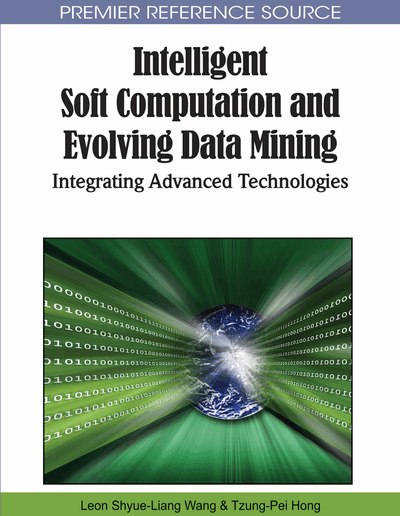# An Intelligent Data Mining System Through Integration Of Electromagnetism-Like Mechanism And Fuzzy Neural Network

Peitsang Wu (I-Shou University, Taiwan, R.O.C.) and Yung-Yao Hung (I-Shou University, Taiwan, R.O.C.)
Copyright: © 2010 |Pages: 24
DOI: 10.4018/978-1-61520-757-2.ch004
OnDemand PDF Download:
Available
\$37.50
No Current Special Offers

## Abstract

In this chapter, a meta-heuristic algorithm (Electromagnetism-like Mechanism, EM) for global optimization is introduced. The Electromagnetism-like mechanism simulates the electromagnetism theory of physics by considering each sample point to be an electrical charge. The EM algorithm utilizes an attraction-repulsion mechanism to move the sample points towards the optimum. The electromagnetism-like mechanism (EM) can be used as a stand-alone approach or as an accompanying algorithm for other methods. Besides, the electromagnetism-like mechanism is not easily trapped into local optimum. Therefore, the purpose of this chapter is using the electromagnetism-like mechanism (EM) to develop an electromagnetism-like mechanism based fuzzy neural network (EMFNN), and employ the EMFNN to train fuzzy if-then rules.
Chapter Preview
Top

## Introduction

The six generation systems (SGS) are constituted by fuzzy logic, neural networks and genetic algorithms (Wang, 1999). During the last decades, there are a lot of researchers employed the above methods on their researches (Buckley and Hayashi, 1992, 1994; Ishibuchi et al., 1992, 1993, 1995; Lin and Lu, 1995). These studies were focus on not only the modification of architectures but also the improvement of performances. Many investigations for SGS werefocus on the optimization problems (Aliev et al., 2001; Goldberg, 1989). Moreover, the merging of fuzzy logic, neural networks and genetic algorithms was also an interesting field (Kuo et al., 1999, 2001, 2002; Shapiro, 2002).

Fuzzy logic has been shown highly promising performance in the area of control (Fukuda and Shibata, 1992; Jang, 1991, 1992, 1993; Lin, 1991, 1995). Fuzzy neural networks (FNN), associates the fuzzy logic with neural networks, has been used to learn the fuzzy if-then rules. There are many literatures that have addressed the architectures of fuzzy neural networks (Gupta and Knopf, 1990; Ishibuchi et al., 1992, 1993, 1995; Takagi and Hayashi, 1991). And each of them has different capability for problem solving. Since the experts’ knowledge is quite subjective, the fuzzy logic attempts to capture it. The fuzzy neural network is used to learn fuzzy IF-THEN rules which can represent the qualitative factors. Recently, the genetic algorithm combined with fuzzy neural network has been proposed (Buckley, 1996; Kuo et al., 2001), and the training results were better than those of the conventional fuzzy neural network.

The Electromagnetism-like Mechanism (EM) is a new meta-heuristic algorithm developed for global optimization (Birbil and Fang, 2003). The Electromagnetism-like Mechanism simulates the electromagnetism theory of physics by considering each sample point to be an electrical charge. The algorithm utilizes an attraction-repulsion mechanism to move the sample points towards the optimality. The EM-like algorithm has been tested and verified to prove it can be converged rapidly (in terms of the number of function evaluations) to the global optimum, and to produce highly efficient results for those problems with varying degree of difficulty (Birbil and Fang, 2003; Birbil et al, 2005).

The original EM was revised by Birbil et al. (2005) in order to make it convergent and after some modifications in the original EM, they proved that their new revised EM exhibits global convergence with probability one. Yang (2002) was the first to use the electromagnetism algorithm for training neural network with great saving on the computation memories and training time. The results indicated that the electromagnetism algorithm performed much better than the genetic algorithm in finding optimal solution globally (Wu et al., 2004).

## Complete Chapter List

Search this Book:
Reset